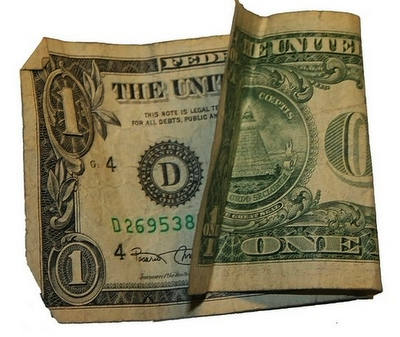## Sunday, November 20, 2011■Three men decided to split the cost of a hotel room. The hotel manager gave them a price of $30. ■The men split the bill evenly, each paying$10, and went to their room. However, the hotel manager realized that it was a Wednesday night, which meant the hotel had a special: rooms were only $25. He had overcharged them$5.

■He called the bellboy, gave him five one-dollar bills and told him to return it to the men.

■When the bellboy explained the situation to the men, they were so pleased at the honesty of the establishment that they promptly tipped the bellboy $2 of the$5 he had returned and each kept $1 for himself. ■So each of the three men ended up paying$9 (their original $10, minus$1 back) totaling $27, plus$2 for the bellboy which makes $29. ■So where is the missing dollar? #### 5 comments: Ken Adams said... It's not missing. The men each paid$25/3 for the room, and $2/3 for a tip to the bellboy - ($25+$2)/3 =$27/3 = $9 for each man. The had each initially paid$10, so their change back of $1 each works out correctly. Mudge said... Way too easy, Hammer. And nice try Ken, but wrong. As anyone with just half a brain (or less, just have to meet the qualifications of a liberal) could tell you, clearly, the men had not sufficiently redistributed their wealth so Obama took it to give to the SEIU so they could fight for bellboy justice and get him more votes. "The Hammer" said... I beg to differ Sir: z = x + iy = |z| (\cos \phi + i\sin \phi ) = r e^{i \phi} \ \bar{z} = x - iy = |z| (\cos \phi - i\sin \phi ) = r e^{-i \phi} where x = \mathrm{Re}\{z\} \, the real part y = \mathrm{Im}\{z\} \, the imaginary part r = |z| = \sqrt{x^2+y^2} the magnitude of z \phi = \arg z = \, atan2(y, x) and furthermore you clearly didn't consider: whereas$1= \cos x\cdot \cos y & = \frac{(e^{ix}+e^{-ix})}{2} \cdot \frac{(e^{iy}+e^{-iy})}{2} \\ & = \frac{1}{2}\cdot \frac{e^{i(x+y)}+e^{i(x-y)}+e^{i(-x+y)}+e^{i(-x-y)}}{2} \\ & = \frac{1}{2} \left[ \{ \frac{e^{i(x+y)} + e^{-i(x+y)}}{2} }_{\cos(x+y)} + \{ \frac{e^{i(x-y)} + e^{-i(x-y)}}{2} }_{\cos(x-y)}.

Mudge said...

Hammer - you forgot to adjust for leap seconds. Otherwise, your calculations are spot on.

Mudge said...

Hammer - you forgot to adjust for leap seconds. Otherwise, your calculations are spot on.

Home